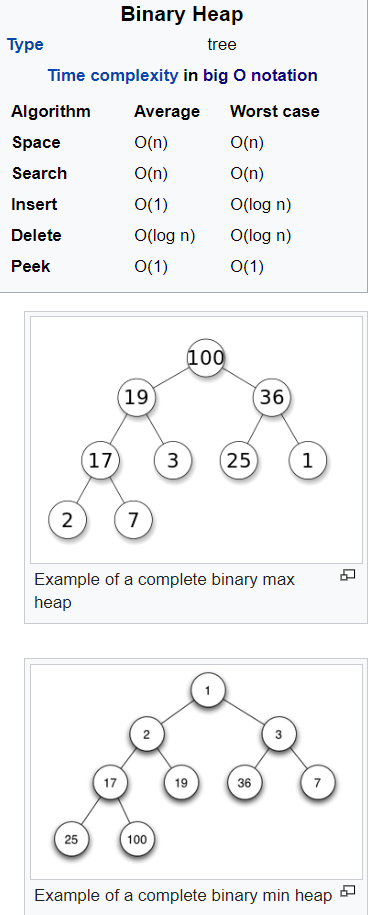# binary heapbinary heap is a heap data structure that takes the form of a binary tree. Binary heaps are a common way of implementing priority queues.:162–163 The binary heap was introduced by J. W. J. Williams in 1964, as a data structure for heapsort.

A binary heap is defined as a binary tree with two additional constraints:

• Shape property: a binary heap is a complete binary tree; that is, all levels of the tree, except possibly the last one (deepest) are fully filled, and, if the last level of the tree is not complete, the nodes of that level are filled from left to right.
• Heap property: the key stored in each node is either greater than or equal to (≥) or less than or equal to (≤) the keys in the node’s children, according to some total order.

Heaps where the parent key is greater than or equal to (≥) the child keys are called max-heaps; those where it is less than or equal to (≤) are called min-heaps. Efficient (logarithmic time) algorithms are known for the two operations needed to implement a priority queue on a binary heap: inserting an element, and removing the smallest or largest element from a min-heap or max-heap, respectively. Binary heaps are also commonly employed in the heapsort sorting algorithm, which is an in-place algorithm because binary heaps can be implemented as an implicit data structure, storing keys in an array and using their relative positions within that array to represent child-parent relationships.

#### insert

1. Add the element to the bottom level of the heap at the most left.
2. Compare the added element with its parent; if they are in the correct order, stop.
3. If not, swap the element with its parent and return to the previous step.

#### Remove

1. Replace the root of the heap with the last element on the last level.
2. Compare the new root with its children; if they are in the correct order, stop.
3. If not, swap the element with one of its children and return to the previous step. (Swap with its smaller child in a min-heap and its larger child in a max-heap.)

#### Heap in C++

You can create with `std::make_heap` a heap. You can push with `std::push_heap` new elements on the heap. On the contrary, you can pop the largest element with `std::pop_heap` from the heap. Both operations respect the heap characteristics. `std::push_heap` moves the last element of the range on the heap; `std::pop_heap` moves the biggest element of the heap to the last position in the range. You can check with `std::is_heap` if a range is a heap. You can determine with `std::is_heap_until` until which position the range is a heap. `std::sort_heap` sorts the heap.

``````std::vector<int> vec{4, 3, 2, 1, 5, 6, 7, 9, 10};
std::make_heap(vec.begin(), vec.end());
for (auto v: vec) std::cout << v << " ";                   // 10 9 7 4 5 6 2 3 1
std::cout << std::is_heap(vec.begin(), vec.end());         // true

vec.push_back(100);
std::cout << std::is_heap(vec.begin(), vec.end());         // false
std::cout << *std::is_heap_until(vec.begin(), vec.end());  // 100
for (auto v: vec) std::cout << v << " ";                   // 10 9 7 4 5 6 2 3 1 100

std::push_heap(vec.begin(), vec.end());
std::cout << std::is_heap(vec.begin(), vec.end());        // true
for (auto v: vec) std::cout << v << " ";                  // 100 10 7 4 9 6 2 3 1 5

std::pop_heap(vec.begin(), vec.end());
for (auto v: vec) std::cout << v << " ";                  // 10 9 7 4 5 6 2 3 1 100
std::cout << *std::is_heap_until(vec.begin(), vec.end()); // 100

vec.resize(vec.size()-1);
std::cout << std::is_heap(vec.begin(), vec.end());        // true
std::cout << vec.front() << std::endl;                    // 10``````